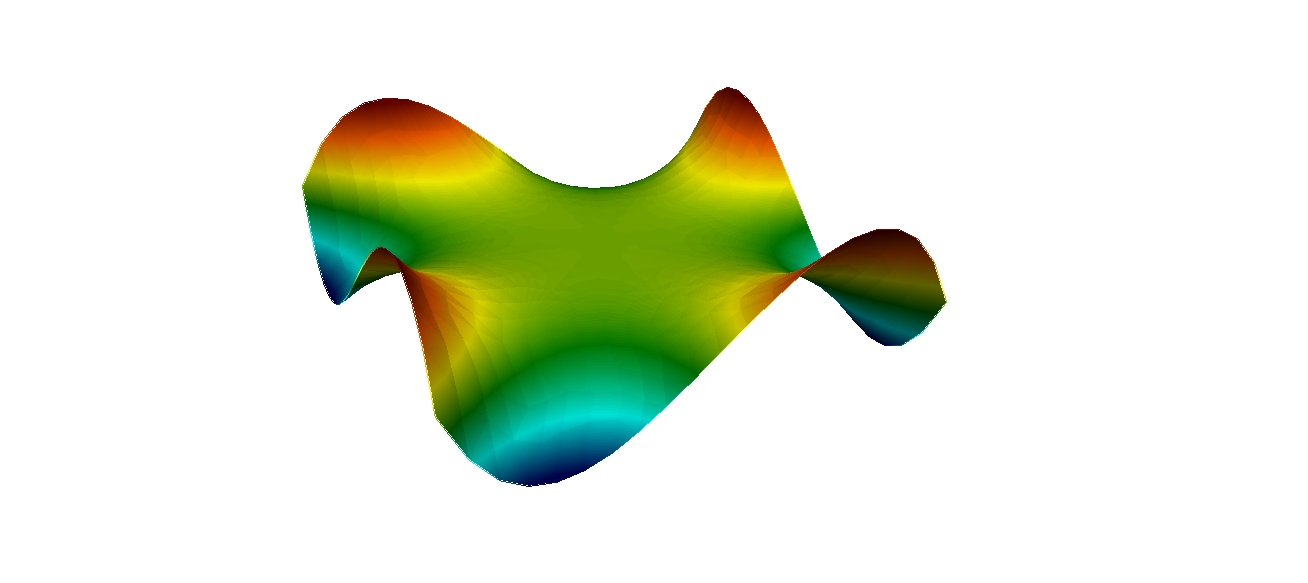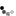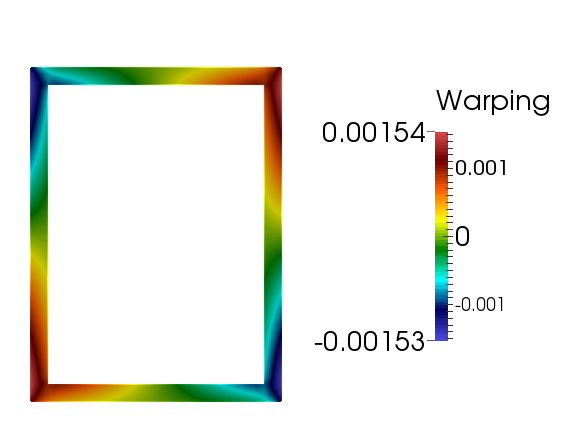## TOBECS

#### TOol for BEam Cross Sectional analysis###### Institute of Information and Communication Technologies
TOBECS computes numerically the cross sectional properties of given 2D section which represents the cross section of a beam. These coefficients are used at the PDE which describes the equation of motion of beams with arbitrary cross sections.

### Cross sectional modelling of beams

Beams are basic structural elements which have applications in many branches of industry. Very often these structures are with non-symmetrical cross sections. Some typical examples include wind turbine blades, bridges, aircraft wings, helicopter blades, etc.

The computation of the cross-sectional properties consists of numerical solution of the elliptic partial differential equation, which defines the warping function, and numerical integration of all cross sectional properties which appear at the equation of motion of beams. The obtained warping function and the computed cross sectional coefficients are suitable for solid, open and closed thin-walled cross sections of arbitrary geometry.

Details about the numerical computation of the warping function and the derivation of the beam equation of motion can be found in the following paper:

S. Stoykov, E. Manoach, S. Margenov, An efficient 3D numerical beam model based on cross sectional analysis and Ritz approximations, ZAMM - Journal of Applied Mathematics and Mechanics 96 (2016) 791-812.

Upload your own mesh, TOBECS will compute the cross sectional properties and generate a vtk file format which represents the warping function for the given cross section. The tool works with msh file formats (generated by Gmsh) and uses linear triangular elements. The mesh should be in xy plane.computing...

The tool gives the coordinates of the twist centre and the centroid, and the following cross sectional properties:

1.$A = \int_{\Omega}d\Omega$ Area [m2]
2.$I_{x_c} = \int_{\Omega}(y-y_c)^2d\Omega$ Second moment of area about x axis with respect to the centroid [m4]
3.$I_{y_c} = \int_{\Omega}(x-x_c)^2d\Omega$ Second moment of area about y axis with respect to the centroid [m4]
4.$I_{x_c y_c} = \int_{\Omega}(x-x_c)(y-y_c)d\Omega$ Product moment of area with respect to the centroid [m4]
5.$C_{\omega} = \int_{\Omega}\psi^2d\Omega$ Warping constant [m6]
6.$I_t = \int_{\Omega}\Big( x^2+y^2+x\frac{\partial \psi}{\partial y} - y\frac{\partial \psi}{\partial x}\Big)d\Omega$ Torsional constant [m4]
7.$S_x = \int_{\Omega}yd\Omega$ First moment of area about x axis [m3]
8.$S_y = \int_{\Omega}xd\Omega$ First moment of area about y axis [m3]
9.$I_x = \int_{\Omega}y^2d\Omega$ Second moment of area about x axis [m4]
10.$I_y = \int_{\Omega}x^2d\Omega$ Second moment of area about y axis [m4]
11.$I_{xy} = \int_{\Omega}xyd\Omega$ Product moment of area [m4]
12.$I_S = \int_{\Omega}\Big( x^2+y^2 \Big)\Omega$ Polar moment of area [m4]
13.$I_R = \int_{\Omega}\Big( x^2+y^2 \Big)^2\Omega$ Fourth moment of area [m6]

### Examples

Example input files (in msh format) and the output vtk files are given here.

• #### I cross section$I section geom$$I section$
• #### Thin-walled cross section$I section geom$$thin-walled$
• #### NACA 63-215 cross section$NACA 63-215$$NACA 63-215$

Tobecs was developed at Institute of Information and Communication Technologies, Bulgarian Academy of Sciences. If you have any questions, comments or suggestions, please contact Dr. Stoykov (Email: stoykov@parallel.bas.bg).